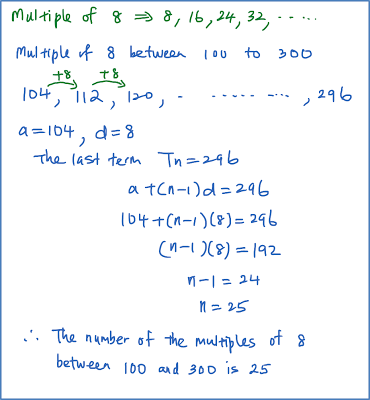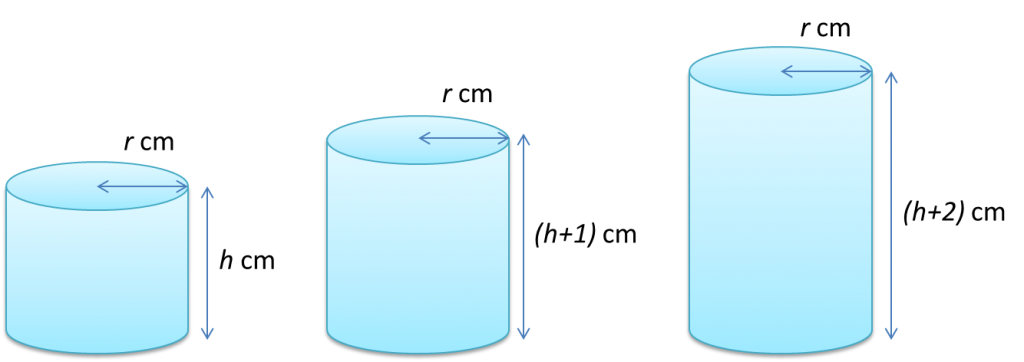# 1.3.2 Arithmetic Progressions, SPM Practice (Paper 1)

Question 6:
Find the number of the multiples of 8 between 100 and 300.

Solution:Question 7:
Find the sum of all the multiples of 7 between 100 and 500.

Solution:Question 8:
If are the first three terms of a progression, show that it forms an arithmetic progression.

Solution:Question 9:Show that the volumes of the cylinders in the above diagram form an arithmetic progression and state its common difference.

Solution:Question 10:
The sequence –11, –5, 1,… is an arithmetic progression. State the three consecutive terms of this arithmetic progression where the sum of these three terms is 93.

Solution:### 1 thought on “1.3.2 Arithmetic Progressions, SPM Practice (Paper 1)”

1.i cant open the next aritmetic progressions